Chapter 2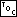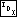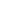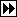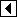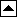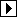#### Section 2.1

1. If the table is not a dictionary, then we can just include a count along with each item recording the number of copies of it that would otherwise be included in the table. If the table is a dictionary, then each data item can include a single key and possibly multiple values.

#### Section 2.2

1. Only macro parameter names can safely appear prefixless. Macro parameter names are significant only in a scope from their declaration to the end of the macro definition. Macro parameters may even be named as otherwise reserved C keywords such as int and while, although this is a bad idea.

The main reason that the other kinds of identifiers must be prefixed is the possibility of a macro having the same name. A surprise macro expansion in the midst of a function prototype can lead to puzzling compiler diagnostics.

2. The capitalized equivalent is ERR_, which is a reserved identifier. All identifiers that begin with an uppercase E followed by a digit or capital letter are reserved in many contexts. It is best to avoid them entirely. There are other identifiers to avoid, too. The article cited below has a handy list.

#### Section 2.3

1. C does not guarantee that an integer cast to a pointer and back retains its value. In addition, there's a chance that an integer cast to a pointer becomes the null pointer value. This latter is not limited to integers with value 0. On the other hand, a nonconstant integer with value 0 is not guaranteed to become a null pointer when cast.

Such a technique is only acceptable when the machine that the code is to run on is known in advance. At best it is inelegant. At worst, it will cause erroneous behavior.

See also:  [Summit 1999], section 5; [ISO 1990], sections 6.2.2.3 and 6.3.4; [ISO 1999], section 6.3.2.3.

2. This definition would only cause problems if the subtraction overflowed. It would be acceptable if it was known that the values to be compared would always be in a small enough range that overflow would never occur.

Here are two more “clever” definitions for compare_ints() that work in all cases:

```/* Credit: GNU C library reference manual. */
int compare_ints (const void *pa, const void *pb, void *param) {
const int *a = pa;
const int *b = pb;

return (*a > *b) - (*a < *b);
}
```
```int compare_ints (const void *pa, const void *pb, void *param) {
const int *a = pa;
const int *b = pb;

return (*a < *b) ? -1 : (*a > *b);
}
```

3. No. Not only does strcmp() take parameters of different types (const char *s instead of const void *s), our comparison functions take an additional parameter. Functions strcmp() and compare_strings() are not compatible.

4.

```int compare_fixed_strings (const void *pa, const void *pb, void *param) {
return memcmp (pa, pb, *(size_t *) param);
}
```

5a. Here's the blow-by-blow rundown:

• Irreflexivity: a == a is always true for integers.
• Antisymmetry: If a > b then b < a for integers.
• Transitivity: If a > b and b > c then a > c for integers.
• Transitivity of equivalence: If a == b and b == c, then a == c for integers.

5b. Yes, strcmp() satisfies all of the points above.

5c. Consider the domain of pairs of integers (x0,x1) with x1 >= x0. Pair x, composed of (x0,x1), is less than pair y, composed of (y0,y1), if x1 < y0. Alternatively, pair x is greater than pair y if x0 > y1. Otherwise, the pairs are equal.

This rule is irreflexive: for any given pair a, neither a1 < a0 nor a0 > a1, so a == a. It is antisymmetic: a > b implies a0 > b1, therefore b1 < a0, and therefore b < a. It is transitive: a > b implies a0 > b1, b > c implies b0 > c1, and we know that b1 > b0, so a0 > b1 > b0 > c1 and a > c. It does not have transitivity of equivalence: suppose that we have a == (1,2), b == (2,3), c == (3,4). Then, a == b and b == c, but not a == c.

A form of augmented binary search tree, called an “interval tree”, can be used to efficiently handle this data type. The references have more details.

6a. !f(a, b) && !f(b, a) and !f(a, b) && f(b, a).

6b.

```static int bin_cmp (const void *a, const void *b, void *param, bst_comparison_func tern) {
return tern (a, b, param) < 0;
}
```

6c. This problem presents an interesting tradeoff. We must choose between sometimes calling the comparison function twice per item to convert our >= knowledge into > or ==, or always traversing all the way to a leaf node, then making a final call to decide on equality. The former choice doesn't provide any new insight, so we choose the latter here.

In the code below, p traverses the tree and q keeps track of the current candidate for a match to item. If the item in p is less than item, then the matching item, if any, must be in the left subtree of p, and we leave q as it was. Otherwise, the item in p is greater than or equal to p and then matching item, if any, is either p itself or in its right subtree, so we set q to the potential match. When we run off the bottom of the tree, we check whether q is really a match by making one additional comparison.

```void *bst_find (const struct bst_table *tree, const void *item) {
const struct bst_node *p;
void *q;

assert (tree != NULL && item != NULL);

p = tree->bst_root;
q = NULL;
while (p != NULL)
if (!bin_cmp (p->bst_data, item, tree->bst_param, tree->bst_compare))       {
q = p->bst_data;
}

if (q != NULL && !bin_cmp (item, q, tree->bst_param, tree->bst_compare))
return q;
else     return NULL;
}
```

#### Section 2.5

1. It's not necessary, for reasons of the C definition of type compatibility. Within a C source file (more technically, a “translation unit”), two structures are compatible only if they are the same structure, regardless of how similar their members may be, so hypothetical structures struct bst_allocator and struct avl_allocator couldn't be mixed together without nasty-smelling casts. On the other hand, prototyped function types are compatible if they have compatible return types and compatible parameter types, so bst_item_func and avl_item_func (say) are interchangeable.

2. This allocator uses the same function tbl_free() as tbl_allocator_default.

```590. <Aborting allocator 590> =
/* Allocates size bytes of space using malloc().
Aborts if out of memory. */
void *tbl_malloc_abort (struct libavl_allocator *allocator, size_t size) {
void *block;

assert (allocator != NULL && size > 0);

block = malloc (size);
if (block != NULL)
return block;

fprintf (stderr, "out of memory\n");
exit (EXIT_FAILURE);
}

struct libavl_allocator tbl_allocator_abort =   {
tbl_malloc_abort,     tbl_free  };
```

3. Define a wrapper structure with struct libavl_allocator as its first member. For instance, a hypothetical pool allocator might look like this:

```struct pool_allocator   {
struct libavl_allocator suballocator;
struct pool *pool;
};
```

Because a pointer to the first member of a structure is a pointer to the structure itself, and vice versa, the allocate and free functions can use a cast to access the larger struct pool_allocator given a pointer to struct libavl_allocator. If we assume the existence of functions pool_malloc() and pool_free() to allocate and free memory within a pool, then we can define the functions for struct pool_allocator's suballocator like this:

```void *pool_allocator_malloc (struct libavl_allocator *allocator, size_t size) {
struct pool_allocator *pa = (struct pool_allocator *) allocator;
return pool_malloc (pa->pool, size);
}

void pool_allocator_free (struct libavl_allocator *allocator, void *ptr) {
struct pool_allocator *pa = (struct pool_allocator *) allocator;
pool_free (pa->pool, ptr);
}
```

Finally, we want to actually allocate a table inside a pool. The following function does this. Notice the way that it uses the pool to store the struct pool_allocator as well; this trick comes in handy sometimes.

```struct tbl_table *pool_allocator_tbl_create (struct tbl_pool *pool) {
struct pool_allocator *pa = pool_malloc (pool, sizeof *pa);
if (pa == NULL)
return NULL;

pa->suballocator.tbl_malloc = pool_allocator_malloc;
pa->suballocator.tbl_free = pool_allocator_free;
pa->pool = pool;
return tbl_create (compare_ints, NULL, &pa->suballocator);
}
```

#### Section 2.7

1. Notice the cast to size_t in the macro definition below. This prevents the result of tbl_count() from being used as an lvalue (that is, on the left side of an assignment operator), because the result of a cast is never an lvalue.

```591. <Table count macro 591> =
#define tbl_count(table) ((size_t) (table)->tbl_count)
```

This code is included in 15.

Another way to get the same effect is to use the unary + operator, like this:

```#define tbl_count(table) (+(table)->tbl_count)
```

#### Section 2.8

1. If a memory allocation function that never returns a null pointer is used, then it is reasonable to use these functions. For instance, tbl_allocator_abort from Exercise 2.5-2 is such an allocator.

2. Among other reasons, tbl_find() returns a null pointer to indicate that no matching item was found in the table. Null pointers in the table could therefore lead to confusing results. It is better to entirely prevent them from being inserted.

3.

```592. <Table insertion convenience functions 592> =
void *tbl_insert (struct tbl_table *table, void *item) {
void **p = tbl_probe (table, item);
return p == NULL || *p == item ? NULL : *p;
}

void *tbl_replace (struct tbl_table *table, void *item) {
void **p = tbl_probe (table, item);
if (p == NULL || *p == item)
return NULL;
else     {
void *r = *p;
*p = item;
return r;
}
}
```

This code is included in 29, 145, 196, 251, 300, 336, 375, 418, 455, 489, 522, and 554.

#### Section 2.9

1. Keep in mind that these directives have to be processed every time the header file is included. (Typical header file are designed to be “idempotent”, i.e., processed by the compiler only on first inclusion and skipped on any later inclusions, because some C constructs cause errors if they are encountered twice during a compilation.)

```593. <Table assertion function control directives 593> =
/* Table assertion functions. */
#ifndef NDEBUG
#undef tbl_assert_insert
#undef tbl_assert_delete
#else
#define tbl_assert_insert(table, item) tbl_insert (table, item)
#define tbl_assert_delete(table, item) tbl_delete (table, item)
#endif
```

This code is included in 24.

2. tbl_assert_insert() must be based on tbl_probe(), because tbl_insert() does not distinguish in its return value between successful insertion and memory allocation errors.

Assertions must be enabled for these functions because we want them to verify success if assertions were enabled at the point from which they were called, not if assertions were enabled when the table was compiled.

Notice the parentheses around the assertion function names before. The parentheses prevent the macros by the same name from being expanded. A function-like macro is only expanded when its name is followed by a left parenthesis, and the extra set of parentheses prevents this from being the case. Alternatively #undef directives could be used to achieve the same effect.

```594. <Table assertion functions 594> =
#undef NDEBUG
#include <assert.h>

void (tbl_assert_insert) (struct tbl_table *table, void *item) {
void **p = tbl_probe (table, item);
assert (p != NULL && *p == item);
}

void *(tbl_assert_delete) (struct tbl_table *table, void *item) {
void *p = tbl_delete (table, item);
assert (p != NULL);
return p;
}
```

This code is included in 29, 145, 196, 251, 300, 336, 375, 418, 455, 489, 522, and 554.

3. The assert() macro is meant for testing for design errors and “impossible” conditions, not runtime errors like disk input/output errors or memory allocation failures. If the memory allocator can fail, then the assert() call in tbl_assert_insert() effectively does this.

#### Section 2.12

1. Both tables and sets store sorted arrangements of unique items. Both require a strict weak ordering on the items that they contain. libavl uses ternary comparison functions whereas the STL uses binary comparison functions (see Exercise 2.3-6).

The description of tables here doesn't list any particular speed requirements for operations, whereas STL sets are constrained in the complexity of their operations. It's worth noting, however, that the libavl implementation of AVL and RB trees meet all of the STL complexity requirements, for their equivalent operations, except one. The exception is that set methods begin() and rbegin() must have constant-time complexity, whereas the equivalent libavl functions *_t_first() and *_t_last() on AVL and RB trees have logarithmic complexity.

libavl traversers and STL iterators have similar semantics. Both remain valid if new items are inserted, and both remain valid if old items are deleted, unless it's the iterator's current item that's deleted.

The STL has a more complete selection of methods than libavl does of table functions, but many of the additional ones (e.g., distance() or erase() each with two iterators as arguments) can be implemented easily in terms of existing libavl functions. These might benefit from optimization possible with specialized implementations, but may not be worth it. The SGI/HP implementation of the STL does not contain any such optimization.